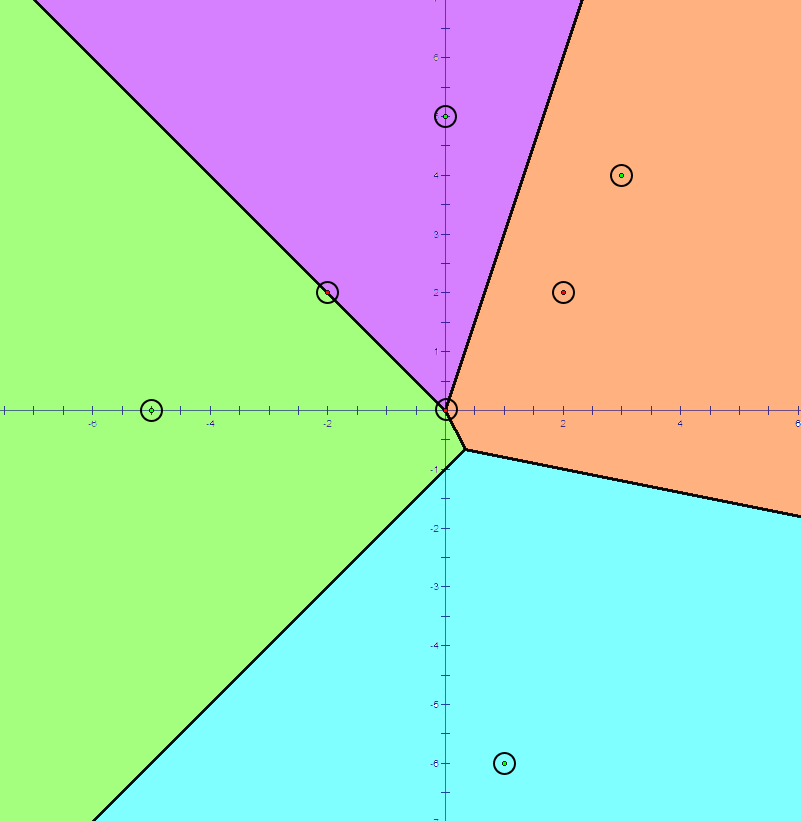시간 제한메모리 제한제출정답맞힌 사람정답 비율
10 초 (추가 시간 없음) 1024 MB124797066.038%

## 문제Figure: Voronoi Diagram of size 4.

In the 2-dimensional Cartesian coordinate system, we define the Voronoi Diagram of a non-empty set of points $S$, as a diagram that divides the plane by the criteria "which point in the set $S$ is closest in this location?". More precisely, the Voronoi diagram of a given non-empty point set $\{P_1,\ P_2,\ \cdots,\ P_n\}$ is a collection of regions: A point $K$ is included in region $i$ if and only if $d(P_i,\ K) \le d(P_j,\ K)$ holds for all $1 \le j \le n$, where $d(X,\ Y)$ denotes the Euclidean distance between point $X$ and $Y$.

For example, in the picture above, every location over the plane is colored by the closest point with such location. The points which belongs to a single region is colored by a light color indicating a region, and the points which belongs to more than one region forms lines and points colored black.

There is an algorithm which computes the Voronoi Diagram in $\mathcal{O}(n \log(n))$, but it is infamous to be very complicated and hard. In fact, we are lenient problem setters, so we set $n \leq 2000$, which means you can solve this task with slower Voronoi Diagram algorithms!

In this task, you should solve the point query problem in Voronoi diagram: in the Voronoi diagram constructed with the set of points $\{P_1,\ P_2,\ \cdots,\ P_n\}$, you should determine which region(s) the point belongs to. More precisely, you will be given $q$ queries of points. For each query point, you should determine the following:

• If it's not included in any region, output NONE.
• If it's included in exactly one region, output REGION X, where X is the index of such region.
• If it's included in exactly two regions, output LINE X Y, where X and Y (X < Y) are the indices of such two regions.
• If it's included in three or more regions, output POINT.

## 입력

In the first line, the number of points consisting Voronoi diagram $n$, and the number of queries $q$ are given. ($3 \le n \le 2, 000,\ 1 \le q \le 250, 000$)

In the $i$th line of next $n$ lines, two integers indicating $x$ and $y$ co-ordinate of $P_i$ are given. These are the points consisting the Voronoi diagram. All $n$ points are distinct. ($|x|,\ |y| \le 10,000$)

In the $j$th line of next $q$ lines, two integers indicating $x$ and $y$ co-ordinate of $Q_j$ are given. For each point $Q_j$, you should determine in which region(s) the given point belongs to. ($|x|,\ |y| \le 10,000$)

## 출력

Output consists of $q$ lines. In the $j$th line, you should print one of following:

• If $Q_j$ is not included in any region, output NONE.
• If $Q_j$ is included in exactly one region, output REGION X, where X is the index of such region.
• If $Q_j$ is included in exactly two regions, output LINE X Y, where X and Y (X < Y) are the indices of such two regions.
• If $Q_j$ is included in three or more regions, output POINT.

## 예제 입력 1

4 3
-5 0
0 5
3 4
1 -6
-2 2
0 0
2 2


## 예제 출력 1

LINE 1 2
POINT
REGION 3


Example is illustrated as a diagram above.